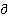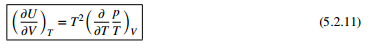### Create an Account

Already have account?

### Forgot Your Password ?

Home / Questions / Use the Helmholtz equation 5211 to show that at constant T the energy of an ideal gas is i...

# Use the Helmholtz equation 5211 to show that at constant T the energy of an ideal gas is independent of volume

Use the Helmholtz equation (5.2.11) to show that, at constant T, the energy of an ideal gas is independent of volume.

(b) Use the Helmholtz equation (5.2.11) to calculate (U/V)T for N moles of a gas using the van der Waals equation.Jun 14 2020 View more View Less

#### Answer (Solved)Subscribe To Get Solution